Rotational motion
Question

# Assume body B to be uniform:Column-AColumn-B (Torque ofnormal reaction on body Babout centre of mass)(a)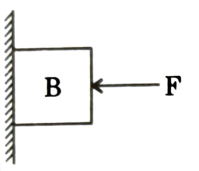(F is directed towards|centre of mass)(p)Clockwise(b)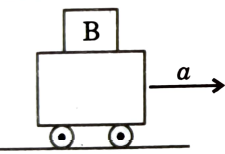(q)Anticlockwise(c)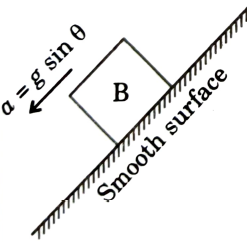(r)Zero  (s)Conclusion cannot be drawnwith the information given

Moderate
Solution

## (a)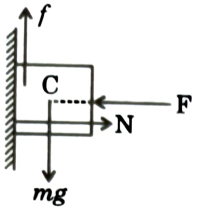To balance torque due to f, N will shift downwards(For rotational equilibrium ${\stackrel{\to }{\mathrm{\tau }}}_{\mathrm{f}}=-{\stackrel{\to }{\mathrm{\tau }}}_{\mathrm{N}}\right)$ (about C)(b)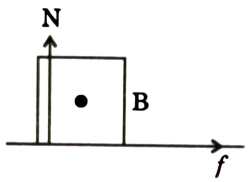Normal will shift towards left.(For rotational equilibrium ${\stackrel{\to }{\mathrm{\tau }}}_{\mathrm{f}}=-{\stackrel{\to }{\mathrm{\tau }}}_{\mathrm{N}}\right)$(c)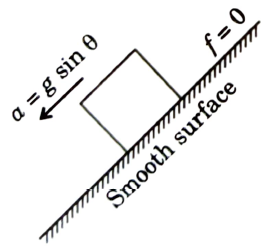Frictionless surface. So, no torque about C.M. Sonormal will not shift and will pass through C.M.

Get Instant Solutions